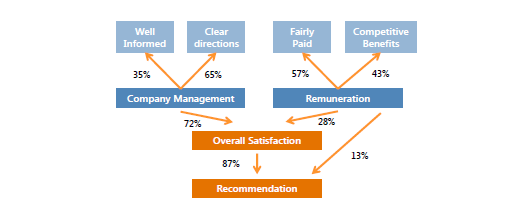Structural Equation Modeling (SEM) is a very general, very powerful multivariate analysis technique that includes a number of other traditional analysis methods as special cases.

### How structural equation modelling works

It effectively includes a whole range of standard multivariate analysis methods, such as regression, factor analysis and analysis of variance. A structural equation model can exist with several regression and factor analysis models, which are estimated simultaneously.

It is a statistical methodology that takes a hypothesis-testing (i.e. confirmatory) approach to the multivariate analysis. SEM tests a theory using survey data, while traditional modeling uses the data to build a model (i.e. exploratory approach). The technique is commonly used for validating models, e.g. a CRM model using survey.